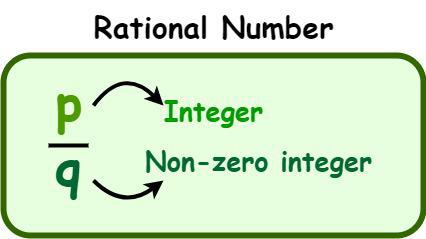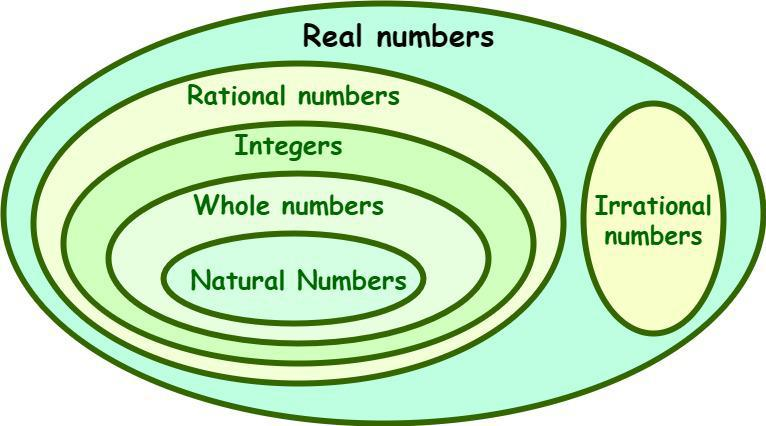GFG App
Open AppBrowser
Continue

# Prove or disprove that if r is rational and s is rational, then r + s must be rational

A rational number is a number that can be expressed in the form of p/q, where p and q are integers, and q is not equal to zero. All natural numbers, whole numbers, integers, fractions of integers, and decimals (terminating decimals and recurring decimals) are subsets of rational numbers. An irrational number is defined as a number that cannot be expressed in the form of fractions. The word “rational” is extracted from the word “ratio”. So, we can conclude that any number that can be expressed as a fraction where the numerator is an integer and the denominator is a non-zero integer, then the number is said to be a rational number. Some of the examples of rational numbers are -5/6, -1/2, 0, 1/2, 2/5, 4/2, etc.### How to identify a rational number?

A rational number can be defined as any fraction that has a non-zero denominator. Go through the following characteristics to identify whether a number is rational or not.

• If a can be expressed in the form of p/q, where p and q are integers, and q is not equal to zero, then the number is rational.
• All natural numbers (N), whole numbers (W), integers (Z), fractions of integers, and decimals (terminating decimals and recurring decimals) are subsets of rational numbers.
• A rational number is a terminating or non-terminating and recurring decimal. For example, 3.6 or 4.181818 are rational numbers.
• If the decimal form of the number is non-recurring or non-terminating, then the number is said to be irrational. For example, √7 is equal to 2.645751311064591…, which is an irrational number as its value is a non-repeating decimal.## Prove or disprove that if r is rational and s is rational, then r + s must be rational.

Solution:

Given that both r and s are rational numbers.

Let r = a/b and s = m/n, where b, n ≠ 0

Now, r + s = (a/b) + (m/n)

= (a×n + b×m)/bn

We know that b, n ≠ 0. So, b × n ≠0.

As a, b, m, and n are integers, so, a×n and b×m are also integers.

Here, (r + s) is in the form of a fraction with a non-zero denominator.

We know that a rational number is a number that can be expressed in the form of p/q, where p and q are integers and q ≠ 0.

So, from the definition of a rational number (r + s) is rational.

Hence proved.

## Solved Examples on Rational Numbers

Example 1: What is the decimal form of the rational number 6/24?

Solution:

The given rational number, 6/24, can be converted into a decimal number by dividing the numerator by the denominator. So, by dividing 6 by 24, we get 0.25. Hence, the decimal value of 6/24 is 0.25.

Example 2: Is √11 a rational number?

Solution:

No, √11 is not a rational number. The value of √11 is 3.3166247903554… It is a non-terminating and non-recurring decimal. So, √11 is an irrational number.

Example 3: Identify the rational numbers among the following numbers: √8, 4/5, √11/3, -2/3, √9, 4.79583152331272…..

Solution:

When a rational number is simplified, it should either be a terminating or non-terminating and recurring decimal. Here, only 4/5, -2/3, and √9 (√9 = 3) are rational numbers among the given numbers.

Example 4: From the given statements choose the correct statement concerning rational numbers.

a) Is every whole number a rational number?

b) Is “e” a rational number?

Solution:

a) We know that whole numbers are a subset of the set of rational numbers. So, every whole number is a rational number. So, this statement is correct.

b) The value of e is 2.718281828459045… It is a non-terminating and non-recurring decimal. So, e is an irrational number. Hence, the given statement is false.

Example 5: Is π a rational number?

Solution:

No, π is not a rational number. The value of π is 3.141592653589793238… It is a non-terminating and non-recurring decimal. So, π is an irrational number.

## FAQs on Rational Numbers

Question 1: What is meant by a rational number? Give some examples of rational numbers.

A rational number is a number that can be expressed in the form of p/q, where p and q are integers, and q is not equal to zero. The set of rational numbers is expressed by the letter “Q” Some of the examples of rational numbers are -5/6, -1/2, 0, 1/2, 2/5, 4/2, etc.

Question 2: What are Irrational Numbers?

An irrational number is defined as a number that cannot be expressed in the form of fractions. The set of irrational numbers is expressed as “Q” Some of the examples of irrational numbers are √2, √3, √7, π, e, and so on.

Question 3: What is the difference between a rational number and an irrational number?

A rational number is a number that can be expressed in the form of p/q, where p and q are integers, and q is not equal to zero. An irrational number is defined as a number that cannot be expressed in the form of fractions. A rational number is a terminating decimal, while an irrational number is non-terminating and non-recurring. Some of the examples of rational numbers are -5/6, -1/2, 0, 1/2, 2/5, 4/2, etc. and the examples of irrational numbers are √2, √3, π, e, etc.

Question 4: Is 0 a rational number?